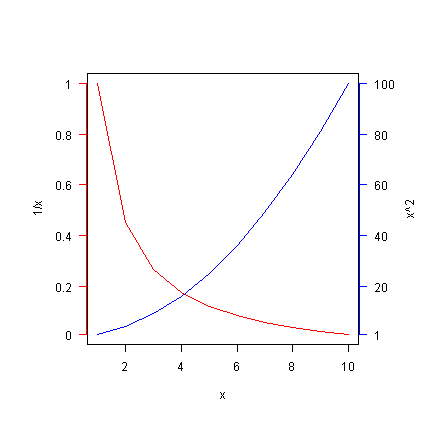## Wednesday, 4 December 2013

### Supplementary functions for plot()

Once a graph is displayed in the graphic device by plot() function, additional information could be inserted and displayed over the graph.

points() function is used in inserting additional points in the displayed graph. This function can display points from a different data source, but will only show those within the x and y limits of the current graph. Hence, it is often used to highlight certain points in the same data set, especially if the data is large and the points are overlapping with each other.

plot(Sepal.Width ~ Sepal.Length, iris, col = "grey", pch = 16)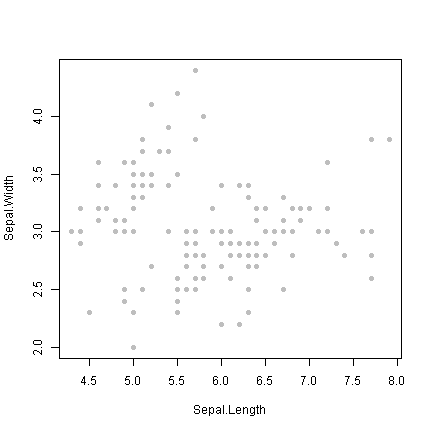The below example identifies setosa species in red colour by using points() function.

Ir1 <- iris[which(iris\$Species == "setosa"), ] plot(Sepal.Width ~ Sepal.Length, iris, col = "grey", pch = 16) points(Ir1\$Sepal.Length, Ir1\$Sepal.Width, col = "red", pch = 16)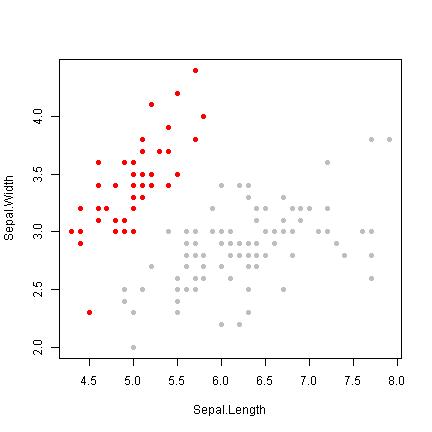lines() function inserts lines over the current graph. This function can be used to join displayed points or insert line of best fit.  The below example shows lines() function used for joining existing plots in the graph. As with plot() function, lines() uses lty to select different line types.
x <- c(1:10) y <- 1/x plot(y ~ x, col = "red", pch = 16) lines(y ~ x, col = "blue", lty = 1)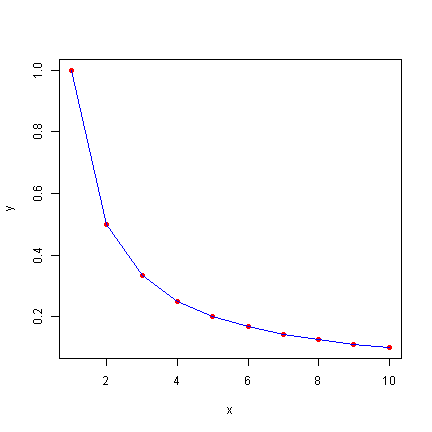The below example shows lines() function used for inserting a line of best fit obtained from a linear regression method (green) and another smoothed line of best fit obtained using lowess() function (red).

LM <- lm(dist ~ speed, cars) plot(cars, col = "steelblue", pch = 16) lines(cars[, "speed"], LM\$fitted.values, col = "green", lty = 1) lines(lowess(cars), col = "red", lty = 1)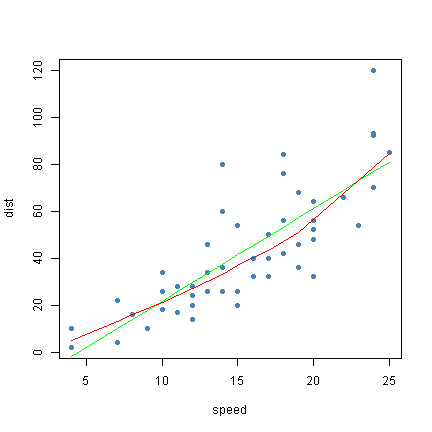Inserting a text on the graph can be done by using text() function. The below example uses the above graph and inserts text labels for the green and red lines of best fit. The position of these inserted texts are assigned using the x,y-coordinates of the graph.

LM <- lm(dist ~ speed, cars) plot(cars, col = "steelblue", pch = 16) lines(cars\$speed, LM\$fitted.values, col = "green", lty = 1) text(cars[nrow(cars), "speed"] - 2, LM\$fitted.value[nrow(cars)] + 2, label = "linear regression", col = "green", cex = 0.7) lines(lowess(cars), col = "red", lty = 1) text(lowess(cars)\$x[nrow(cars)] - 2, lowess(cars)\$y[nrow(cars)] - 17, label = "lowess", col = "red", cex = 0.7)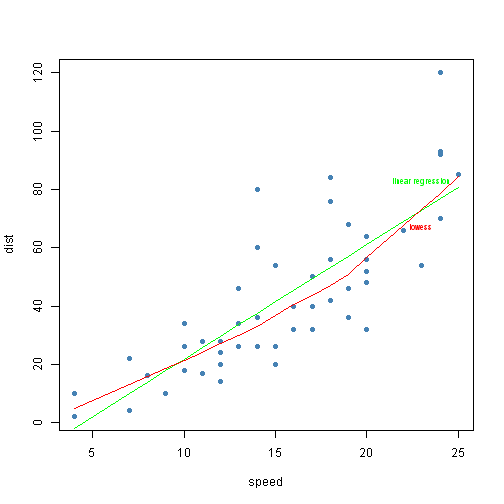text() function can be used to replace plotted points with text labels as shown in the below example.

plot(uptake ~ conc, CO2, type = "n") text(CO2\$conc, CO2\$uptake, label = CO2\$Type, col = as.numeric(factor(CO2\$Treatment)), cex = 0.7)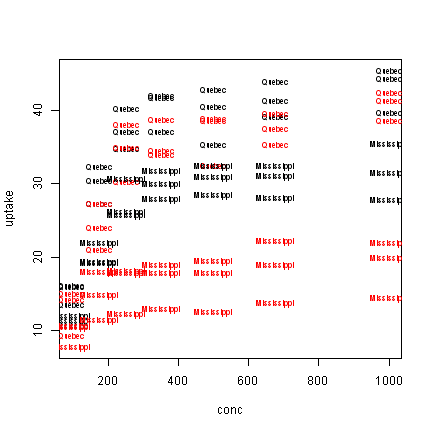The below example shows the use of pos parameter within text() function. This places text labels adjacent to the plotted points, and the positions are assigned by the following numeric values.
1 = below the dot
2 = left side of the dot
3 = above the dot
4 = right side of the dot

plot(uptake ~ conc, CO2, type = "p", col = "steelblue", cex = 1.3) text(CO2\$conc, CO2\$uptake, label = CO2\$Type, col = as.numeric(factor(CO2\$Treatment)), cex = 0.7, pos = 4)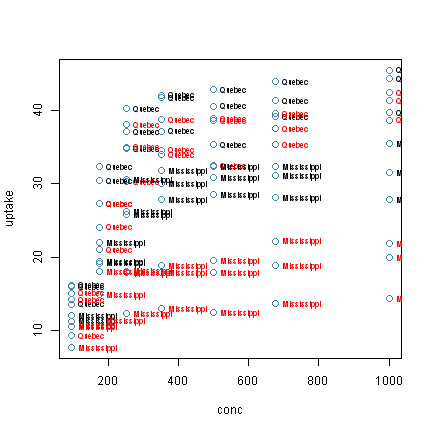mtext() function inserts text labels in the outer margin of the graph. The below is an example.

plot(uptake ~ conc, CO2, type = "p", main = "CO2", col = "steelblue", cex = 1.3) mtext("Carbon Dioxide", 3, line = 0.5, cex = 0.8)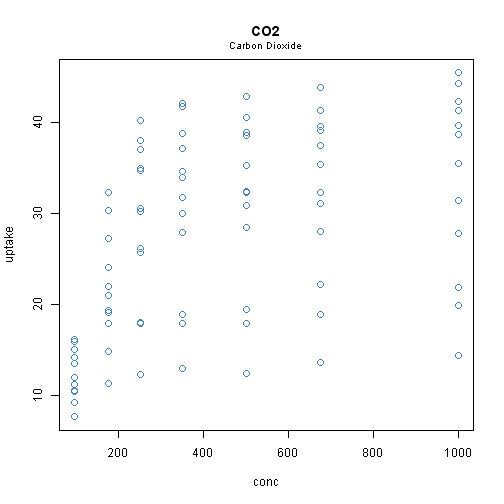Adding a straight line, often used to draw a reference line, on the graph can be done by abline() function. It can assign gradient and intercept to draw a straight line, as in the case of line of best fit obtained from the linear regression method, or assign x or y intercept to draw a vertical or horizontal line.

LM <- lm(dist ~ speed, cars) plot(cars, col = "steelblue", pch = 16) abline(v = 20, lty = 2) text(x = 21, y = 20, label = "vertical line", cex = 0.7) abline(h = 100, lty = 2) text(x = 23, y = 105, label = "horizontal line", cex = 0.7) abline(a = 10, b = 2, col = "red", lty = 3) text(x = 21, y = 56, label = "gradient = 2, intercept = 10", col = "red", cex = 0.7) abline(LM, col = "green", lty = 1) text(x = 22, y = 80, label = "linear regression", cex = 0.7, col = "green")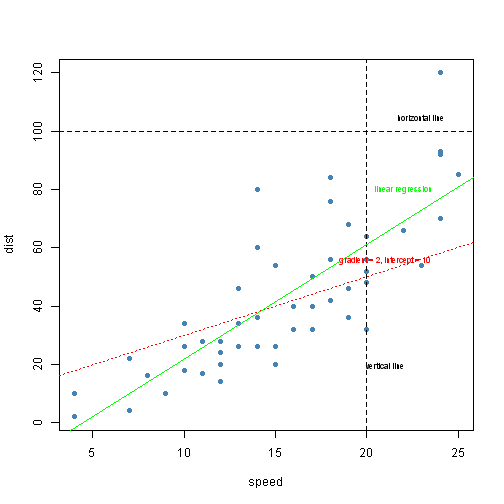When inserting a legend in the graph, legend() function is used followed by an execution of plot() command. The location of legend can be assigned within legend() function either by a x,y-coordinate or by using one of the following text directions.
'top' = at the top of the graph
'right' = right side of the graph
'left' = left side of the graph
'bottom' = at the bottom of the graph
'topright' = at the top right hand corner of the graph
'topleft' = at the top left hand corner of the graph
'bottomright' = at the bottom right hand corner of the graph
'bottomleft' = at the bottom left hand corner of the graph

plot(uptake ~ conc, CO2, col = as.numeric(factor(CO2\$Treatment)), pch = as.numeric(factor(CO2\$Type))) legend("topleft", legend = c(levels(factor(CO2\$Treatment)), levels(factor(CO2\$Type))), col = c(1, 2, "grey", "grey"), pch = c(15, 15, 1, 2), cex = 0.8, pt.cex = 0.8)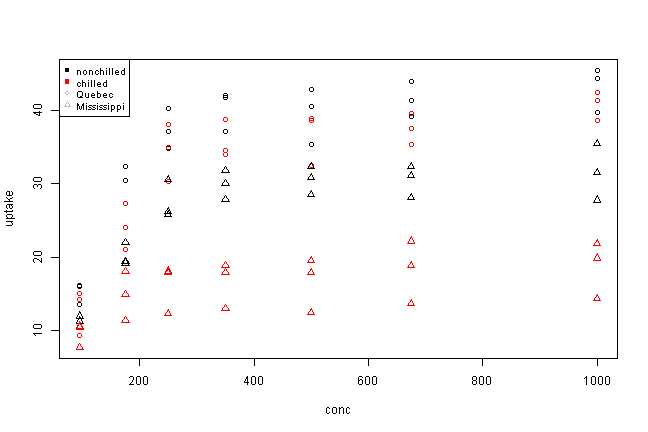This usually places legend inside the graph's plotting region. To place the legend outside the graph's plotting region, we need to set xpd=TRUE and use inset parameter to position the legend. Also, margin need to be set appropriately to allow for the legend to be displayed correctly.

par(mar=c(5,5,3,10), xpd=TRUE)

plot(uptake ~ conc, CO2, col = as.numeric(factor(CO2\$Treatment)), pch = as.numeric(factor(CO2\$Type)))
legend("right", inset=c(-0.2,0), legend = c(levels(factor(CO2\$Treatment)), levels(factor(CO2\$Type))), col = c(1, 2, "grey", "grey"), pch = c(15, 15, 1, 2), cex = 0.8, pt.cex = 0.8)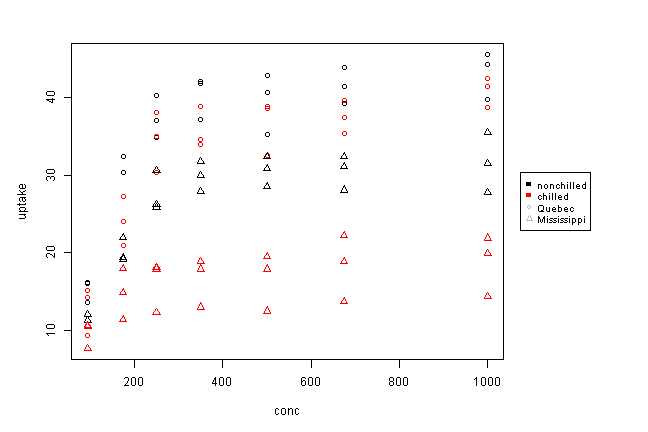An alternative, and perhaps interactive, way of placing legend at the preferred position is to use locator() parameter. This allows the user to click with the mouse a location of the graph where the legend should be inserted. The number assigned in the locator() parameter represents the number of clicks allowed before deactivating the interactive mode. Hence, 1 is usually used for inserting a legend, while other numbers are usually used for inserting text labels.

LM<-lm(dist~speed,cars) plot(cars,col="steelblue",pch=16) lines(cars[,"speed"],LM\$fitted.values,col="green",lty=1) lines(lowess(cars),col="red",lty=1) legend(locator(1),legend=c("LM","lowess"),col=c("green","red"),lty=1) text(locator(4),label="cars",cex=1)

A title and/or subtitle of the graph is usually inserted within plot() function, but for whatever reason, it needs to be inserted after the graph is drawn, title() function is used. This is perhaps useful when plot() function becomes lengthy and complex with all other parameters.

plot(uptake ~ conc, CO2, type = "p") title(main = "CO2 Absoprtion", sub = "Change in Absorption Rate by Concentration")

##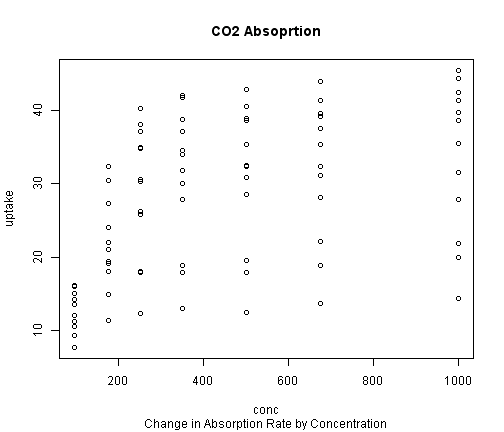To identify the points from the graph, identify() function is used. The user can click, with the mouse, the points on the graph that are interesting, then press esc once finished clicking all the points of interest. The values of those selected points will be displayed on the graph. The below example will show the values of 'Type' variable in CO2 data set when the points are clicked.

plot(uptake~conc,CO2,type="p") identify(CO2[,c("conc","uptake")],label=CO2\$Type)

When you want to join the plotted points to display an area on the graph, ploygon() function is used. The below example displays the area representing the difference between actual values and estimated values obtained by linear regression.

Dat_QNC <- subset(CO2, Plant == "Qn1" & Type == "Quebec" & Treatment == "nonchilled") LM <- lm(uptake ~ conc, Dat_QNC) P <- predict(LM, data.frame(conc = c(160, 770))) A <- rbind(cbind(conc = c(160, 770), uptake = P), Dat_QNC[which(Dat_QNC\$conc >= 160 & Dat_QNC\$conc <= 770), c("conc", "uptake")]) A <- A[order(A\$conc), ] plot(uptake ~ conc, Dat_QNC, type = "l") abline(LM, lty = 3) polygon(A\$conc, A\$uptake, col = "yellow", border = "green")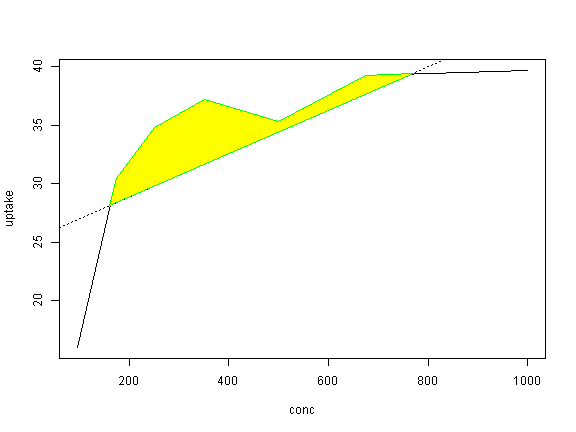Once graph is plotted, additional axis could be inserted using axis() function. This allows inserting axis at all 4 sides of the graph. The below numeric indicators represent the position of the axis.
1 = below the graph
2 = left side of the graph
3 = above the graph
4 = right side of the graph

x <- c(1:10) par(oma = c(1, 2, 1, 3)) plot(1/x ~ x, col = "red", type = "l", lty = 1, yaxt = "n", ylab = "1/x")
par(new = TRUE) plot(x^2 ~ x, col = "blue", type = "l", lty = 1, yaxt = "n", ylab = "") axis(2, at = c(1, seq(20, 100, by = 20)), label = seq(0, 1, by = 0.2), pos = c(0.6, 0), lty = 1, col = "red", las = 2, cex = 0.6) axis(4, at = c(1, seq(20, 100, by = 20)), label = NULL, pos = c(10.4, 0), lty = 1, col = "blue", las = 2, cex = 0.6) mtext("x^2", 4, line = 3)